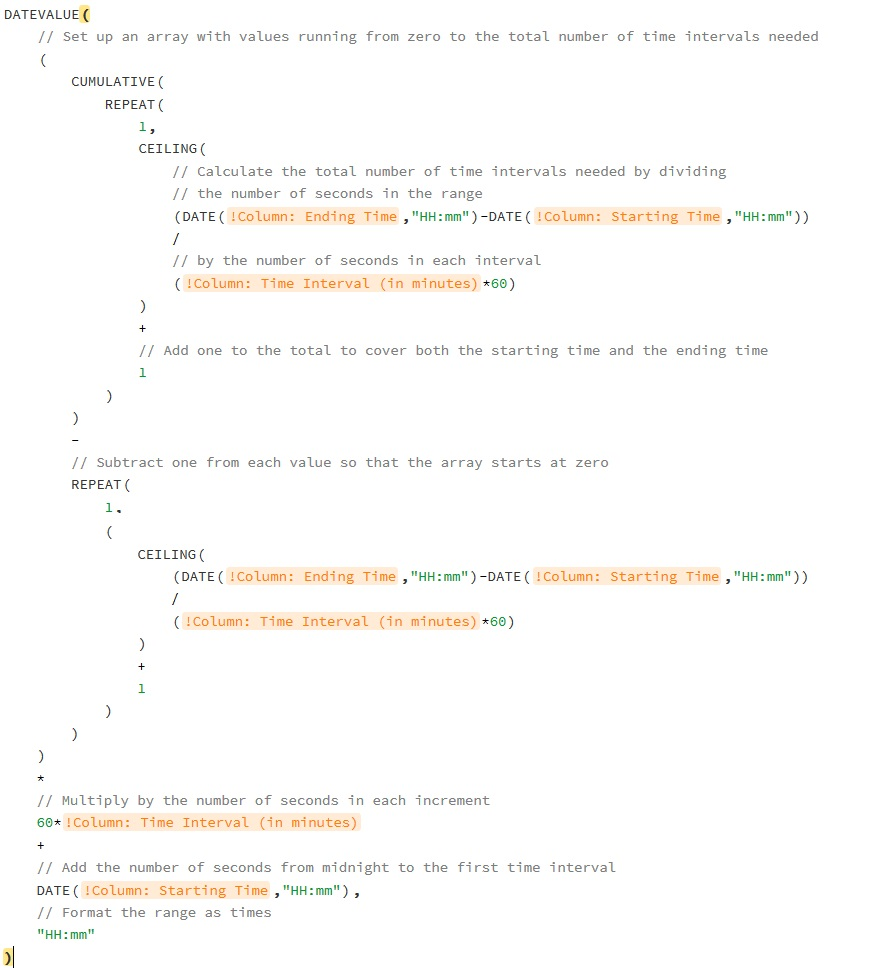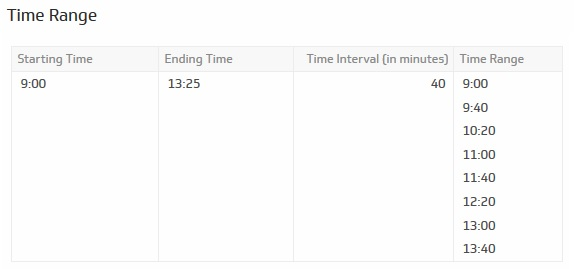Can we have a TIMERANGE function similar to the DATERANGE function?  This would be useful when graphing from data sources that skip entries where the data value is 0, such as a SQL query.

•Janice Janczyn Official comment

Hi Howard, that's a very interesting suggestion. Please feel free to request this capability in Idea Submissions.

Thanks,

Janice

•Jennifer Kirsch

Hi Howard,

You asked about a TIMERANGE function a while ago, so I don't know if you still need it, but here's a formula I just developed to generate time ranges. (It's useful in chart x-axes.)You can hard-code the starting and ending times and the interval length, or you can hide them in table columns, as in this example.And here's the code:

DATEVALUE(
// Set up an array with values running from zero to the total number of time intervals needed
(
CUMULATIVE(
REPEAT(
1,
CEILING(
// Calculate the total number of time intervals needed by dividing
// the number of seconds in the range
(DATE(!Column: Ending Time,"HH:mm")-DATE(!Column: Starting Time,"HH:mm"))
/
// by the number of seconds in each interval
(!Column: Time Interval (in minutes)*60)
)
+
// Add one to the total to cover both the starting time and the ending time
1
)
)
-
// Subtract one from each value so that the array starts at zero
REPEAT(
1,
(
CEILING(
(DATE(!Column: Ending Time,"HH:mm")-DATE(!Column: Starting Time,"HH:mm"))
/
(!Column: Time Interval (in minutes)'*60)
)
+
1
)
)
)
*
// Multiply by the number of seconds in each increment
60*!Column: Time Interval (in minutes)
+
// Add the number of seconds from midnight to the first time interval
DATE(!Column: Starting Time,"HH:mm"),
// Format the range as times
"HH:mm"
)

•DGT Tecnologia

I would like calculate a number of days between  future date with today.

I am use:

COUNT_DAYS(@Resumo,E5;,NOW()) and the result is 19,043

or use

COUNT_DAYS(&Column: Término de contrato,NOW()) and result is 18,754

I know the correct result is 125 because today is 02/20/2022 and my future data is 06/25/2022.

How is the best formula?